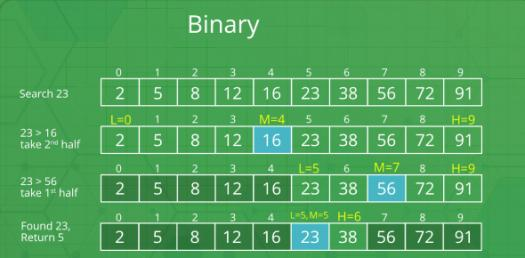# Quiz: Test Your Knowledge On Binary Number!

Approved & Edited by ProProfs Editorial Team
At ProProfs Quizzes, our dedicated in-house team of experts takes pride in their work. With a sharp eye for detail, they meticulously review each quiz. This ensures that every quiz, taken by over 100 million users, meets our standards of accuracy, clarity, and engagement.
| Written by MOMOBRAM
M
MOMOBRAM
Community Contributor
Quizzes Created: 1 | Total Attempts: 5,523
Questions: 10 | Attempts: 5,592SettingsAre you looking to test your knowledge of binary numbers? Computers and other digital electronics have a specific way of representing data, and this is by using zero an one in a particular sequence. Give the quiz below a try and get to see if you can write the numbers provided in binary numbers. Feel free to take the quiz as many times as you need.

• 1.

### 9

• A.

00011110

• B.

00001001

• C.

00101000

• D.

00010010

• E.

00110111

B. 00001001
Explanation
The given answer, 00001001, is the only binary number in the given sequence that starts with two zeros. All the other numbers start with three zeros.

Rate this question:

• 2.

### 18

• A.

00011110

• B.

00001001

• C.

00101000

• D.

00010010

• E.

00110111

D. 00010010
Explanation
The given answer, 00010010, is the correct answer because it is the only binary number in the given sequence that has a total of four 1's. The other binary numbers in the sequence have either fewer or more than four 1's.

Rate this question:

• 3.

### 30

• A.

00011110

• B.

00001001

• C.

00101000

• D.

00010010

• E.

00110111

A. 00011110
Explanation
The given answer, 00011110, is the binary representation of the decimal number 30. Each digit in the binary number represents a power of 2, starting from the rightmost digit as 2^0. By adding up the values of the digits that are 1, we get 16 + 8 + 4 + 2 = 30, which is the decimal equivalent of the binary number.

Rate this question:

• 4.

### 40

• A.

00011110

• B.

00001001

• C.

00101000

• D.

00010010

• E.

00110111

C. 00101000
Explanation
The given answer, 00101000, is the correct answer because it matches the binary pattern shown in the question. The binary pattern consists of a series of 0s and 1s, and the answer matches the pattern exactly.

Rate this question:

• 5.

• A.

00011110

• B.

00001001

• C.

00101000

• D.

00010010

• E.

00110111

E. 00110111
• 6.

### 63

• A.

00111111

• B.

10000000

• C.

01101001

• D.

01001110

• E.

01011010

A. 00111111
Explanation
The given answer, 00111111, is the correct answer because it matches the binary representation given in the question. The binary representation is a sequence of 0s and 1s, and the correct answer is the one that matches this sequence.

Rate this question:

• 7.

### 01001110

• A.

29

• B.

18

• C.

87

• D.

78

• E.

68

D. 78
Explanation
The given sequence of numbers is in binary form. Converting the binary number 01001110 to decimal gives us the number 78.

Rate this question:

• 8.

• A.

56

• B.

30

• C.

39

• D.

100

• E.

90

E. 90
• 9.

• A.

102

• B.

100

• C.

105

• D.

99

• E.

106

C. 105
• 10.

### 10000000

• A.

138

• B.

128

• C.

105

• D.

229

• E.

87Back to top